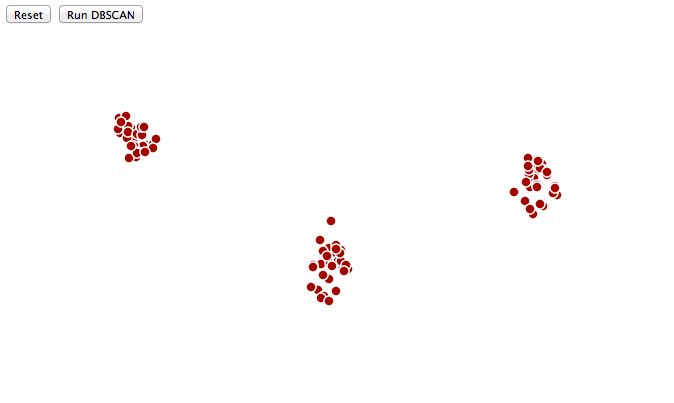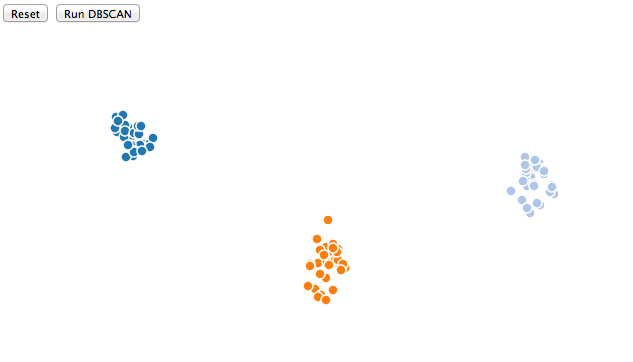Napping Peanut Monsters

# npm

Miss any of our Open RFC calls?Watch the recordings here! »

## jdbscan

1.0.0 • Public • Published

# jDBSCAN

## Description

DBSCAN is a density based clustering algorithm that works by successively growing a cluster from initial seed points . If the density in the circle proximity (which has the radius parameter Eps) of a point is above or equal a threshold level, denoted by the MinPts parameter, the cluster is expanded forward by assigning all the unassigned points in the neighborhood to it. The algorithm then recursively proceeds with the same steps for each of the newly added points to the cluster. Points that will not be assigned to any cluster by the end of this process are labeled as noise.

The current implementation supports only two dimensional data.

The algorithm also works on data points given by GPS coordinates. Moreover, the algorithm can be used on data with a time dimension also. This allows for spatio-temporal clustering.

## Usage

1. Import the script.

`````` <script type="text/javascript" src="jDBSCAN.js"></script>
``````
2. Sample Data Format

#### GPS Data

Where accuracy is given in meters.

### OR

Where timestamp is given by the UNIX timestamp in seconds for the sample point.

1. Run the algorithm. To run the algorithm you need to provide the data along with the eps and minPts parameters. For the traditional DBSCAN the steps are the following:

The distance functions available are: 'EUCLIDEAN', 'HAVERSINE' (for GPS data), 'MANHATTAN'.

Additionally you can provide your own distance function, which must accept at least two parameters (the two points), and passing it to the distance method. The next step is to simply run the clustering algorithm.

In case of spatio-temporal data, as described above, additional parameters must be supplied. Such as time_eps (difference in seconds used as the time equivalent of the distance eps value).

The default time distance function is given by the absolute difference between timestamps. Other functions can be used by passing a function to the time_distance method, it also should accept two objects with a timestamp field.

The remaining steps are similar.

## Example

See example.html, use the console to view the raw input data and raw output.#### After DBSCAN## Keywords

### Install

`npm i jdbscan`

3

1.0.0

MIT

105 kB

12